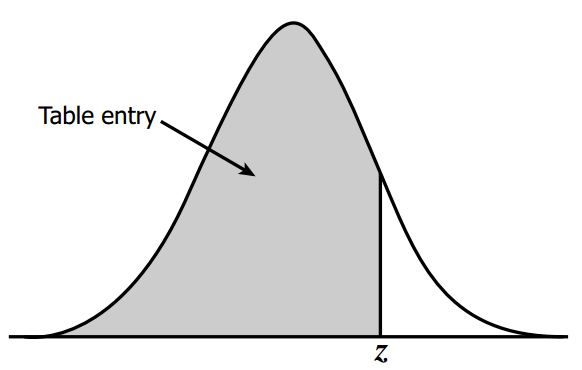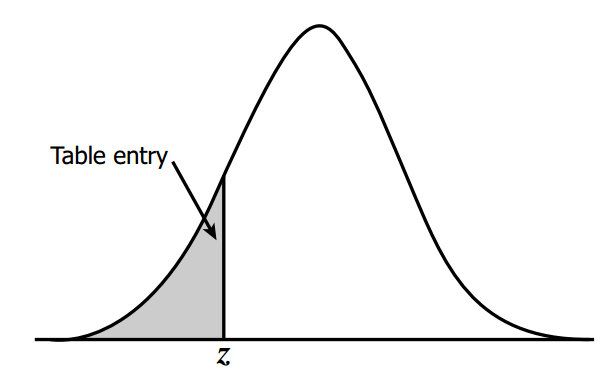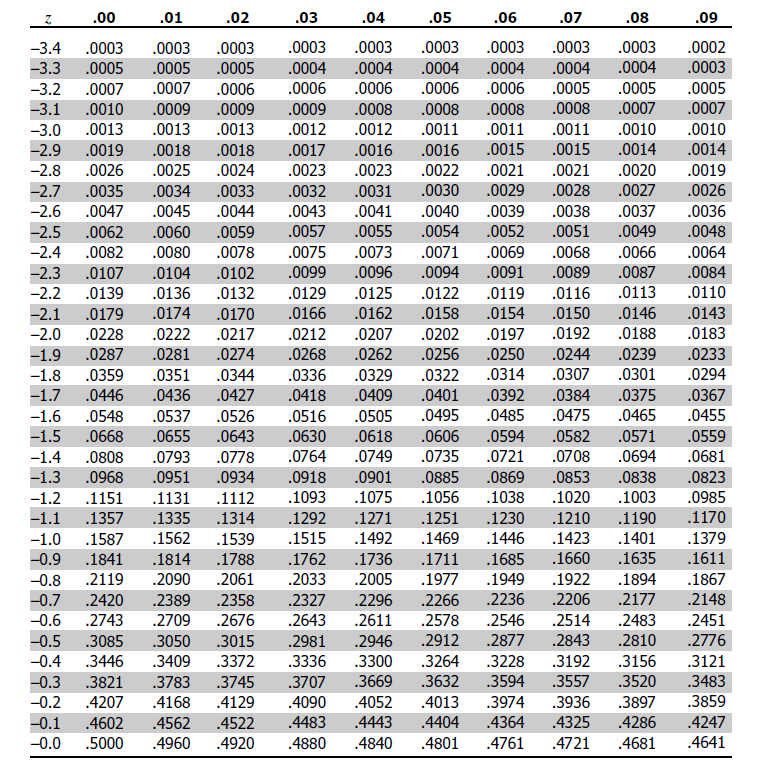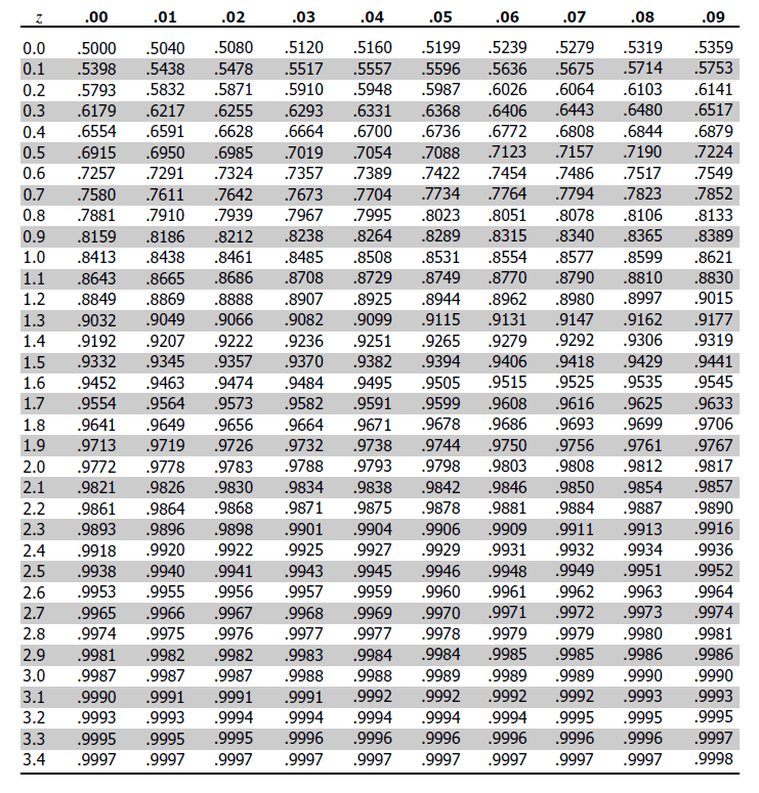# Statistics Module1: Standard Normal Worksheet Problems

Refer to the Standard Normal cumulative probability table below or download the table from  here.Source of table: http://www.z-table.com/

Below is a list of questions based on the Statistics module 1.

Normal Distribution and Z-scoresRefer to the sample table of data sheet of marks of ten students in a test. Assume the marks of the students are normally distributed. Refer to normal probability table for Z scores, they are freely available on internet. Refer to the Module 1 Statistics module before attempting this exercise.

1. Calculate the mean (average) and standard deviation of the sample using excel. Also, calculate the standard deviation using mean method using excel. (69.6,8.66)

2. Person 4 scored 73. What’s the corresponding percentile. The percentile its better than.

(4.27%; 65.29%) Use Norm.dist function in excel.  NORM.DIST(73,69.6,8.65, False)  for the first one and NORM.DIST(73,69.6,8.65, True for second). The solution has been corrected and the second section added.

(NORM.DIST returns cumulative distribution for True and  Probability mass function for False). Refer here.

3. If a person wants to score 80 percentile based on the sample distribution shared, what should be the score in the test? (76.874) Revised this question.

A Math and Verbal test had the following normal distribution. Math N (69, 19) and Verbal N (75,9). Answer the following questions based on the data shared. What’s the mean and standard deviation of the two mentioned distribution?

1. What is the mark of the student who scored the 99th percentile in the math and verbal examination? (M 113.27)
2. What is the score of the student who scored worse than 80 percent of the test-takers in the Verbal examination? Is there a difference between this score from that of the score for a student in Math examination? If so why? (Ans 67.4 Verbal)
3. A case study competition has implemented a rule (a sad rule) that only students among the top ten percent in the examination would be selected for the competition. Based on the sample distribution shared, at what is the cut-off score for both the examinations? (Math 93.3, Verbal: 86.5  Excel function NORM.INV(0.9,69,19); NORM.INV(0.9,75, 9)

For more practice refer to the worksheet second here.

Bernoulli Distribution

In an attempt to prevent Malaria, a non-profit distributes mosquito nets in a village. Consider this an intervention and  a project . The village is under threat of contacting Malaria from other villages as the epidemic is spreading.

Take the prevention of Malaria as a success for this intervention.

After the project is over, the estimate that households don’t have Malaria is 62%. Not having Malaria in a trial is a success. Answer the following questions below.

(Assume that the village never had any mosquito nets and all households received free mosquito nets, and all of them used them during the time of the intervention. Further, there was no case of Malaria among the sampled people both in the control and treatment group before this intervention. During this time Malaria spread from other villages to the present village. Finally, assume that the selection of people is independent and identically distributed. )

1. What’s is the probability that five households randomly sampled at the end of the intervention have Malaria? (at least there is case of one malaria among 5 households).  0.908
2. What is the probability that none of the five sampled households have Malaria? 0.092
3. On average how many people will you sample before you come across as someone who has no Malaria? 1/0.62